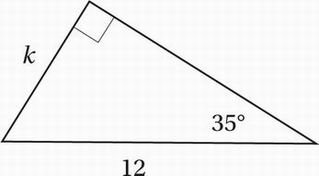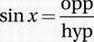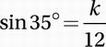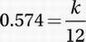# SAT Math Multiple Choice Question 667: Answer and Explanation

### Test Information

Question: 667

7.In the triangle above, what is the value of k? (sin 35° = 0.574, cos 35° = 0.819, tan 35° = 0.700)

• A. 6.00
• B. 6.88
• C. 8.40
• D. 9.83

Explanation:

B

Remember the definitions of the basic trigonometric functions: SOH CAH TOA. Since the "side of interest" (k) is the OPPOSITE side to the given angle (35°), and since we know the length of the HYPOTENUSE (12), we should use SOH.Plug in the values:Substitute sin 35° = 0.574:Multiply by 12:

(12)(0.574) = 6.88 = k